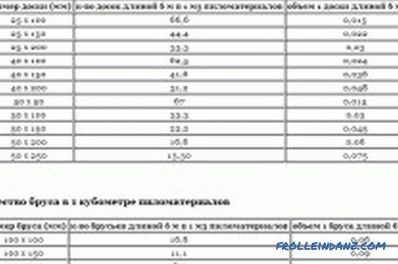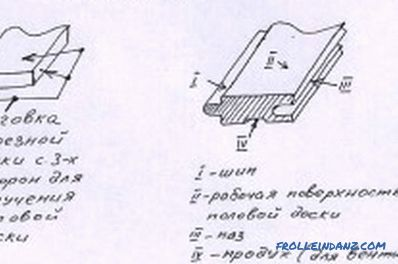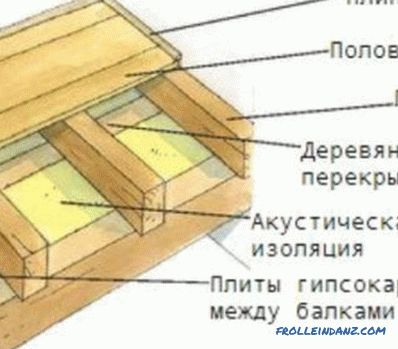# How to calculate the cube of edged and unedged boards: technology

Any construction work begins with the drafting of the project and estimates, which involve the calculation of the amount of building material. The boards, the number of which must also be correctly calculated, are no exception. This will save you from excess cash costs in case of excess or shortage of material.Types of lumber.

The specifics of selling lumber are quite complex, so in some cases even the regulatory authorities cannot assess the accuracy of the release of such products. Unlike most other types of building materials, boards are not sold by weight or by piece, but are calculated in m 3 . Therefore, you need to know how to correctly calculate the cube of the board.

## Technology for calculating the cubic capacity of the board

In some cases, manufacturers supply lumber to the sales market in ready-made packages, attaching a tag to them with the exact volume and price of such products. If there is such a tag, it greatly simplifies the entire calculation process. But this happens very rarely, since usually all measurements are carried out by the storekeeper of the logging company.

The calculation of cubic capacity will directly depend on the degree of wood processing, its variety and type.

Therefore, the edged and unedged boards will be calculated differently. Also, the calculation will depend on the type of wood. So, for hardwood and softwood, you can use both the measurement and multiplication of the dimensions of one element with subsequent multiplication by their number, and the standard - special tables (cubes).

### How to calculate a cube of a cut boardTable of the number of cut boards in 1 cubic meter.

The range of logging companies includes an edging board - one of the most popular types of products, the main feature of which is the processing of lumber from all sides. First, logs are sawn into boards, after which they are further sawn from the sides. Removing the bark can not only facilitate the work with this material and improve its joining, but also protect the wood from harmful microorganisms that eventually hit the bark.

To increase the performance of boards, they are subjected to many processing operations, as a result of which their price will be significantly higher than the uncut option. The cost of building material will depend on its grade, which can be selective, first, second, third and fourth. The higher the grade, the higher will be the cost of the material.

There is nothing difficult in determining the required volume of a cut board. This operation does not take you much time and effort. The calculation will be made according to the formula: V = l * h * a, where: V is the volume of lumber, l is the length, h is the height, and a is the width. For example, it is required to calculate the volume of one board with the size of 20x100x600 mm. Substituting these values ​​into the formula, we get: V = 20 * 100 * 600 = 0.02 * 0.1 * 6 = 0.012 m 3 . With this value, you can calculate the number of such elements in 1 m 3 . To do this, 1 m 3 should be divided by the volume of one element. For the given example in 1 m 3 there will be: 1 / 0,012 = 83.3 or 83 pcs.

To simplify the calculations, you can use the cutting edge of the plank presented in Table 1.

Table 1

 Size of cut timber, mm The number of elements with a length of 6 m in 1 m 3 The volume of one board, m 3 25x100 66.67 0.015 25x150 44.44 0.0225 25x200 33.33 0.03 40x100 41.67 0.024 40x200 20.83 0.048 50x100 33.33 0.03 50x200 16.67 0.06

### How to calculate a cube of uncut materialLayout of the floor board device.

Unedged sawn timber is produced by longitudinal sawing logs without further processing on the sides. In this regard, it is very difficult or even impossible to calculate the cubic capacity of a certain amount of lumber through the calculation of the volume of one element.

In contrast to the edged board, which has standard dimensions, the unedged material has a standard thickness and length, and the width changes, because it depends on which section of the log this particular element is cut from.

Therefore, the calculation of the cubic capacity in this case will be made according to another principle.

For example, if the task is to sheathe a building, then you should simply find out the total finishing area, then multiply it by the thickness of the sheathing, which will be the required volume of lumber. As an example, the situation will be given when it is necessary to calculate how much an unedged board with a thickness of 25 mm will go to the casing of a rectangular building 7x4 m with a wall height of 3 m.

First you need to calculate the total finishing area, for which the perimeter of the building should be multiplied by the height of the walls: (7 + 7 + 4 + 4) * 3 = 66 m 2 . Then this value is multiplied by the thickness of the timber: 66 * 0.025 = 1.65 m 3 . What width the individual cladding elements will be does not matter, since this does not affect the cubic capacity.

However, the average dimensions of one average board should still be known. Table 2 shows the cubicle unedged timber.

Table 2

 Board thickness with a length of 6 m, mm 50 40 25 Average volume of one element, m 3 0.071 0.05 0.0294

From the data in the table, it can be calculated that with a material thickness of 50 mm, the average width will be equal to 230 mm and at 40 mm - approximately 210 mm, and at 25 mm - 190 mm. This is logical, because thicker boards are made from logs of larger cross sections.

•How to calculate the cost of a bar for a house? 3>

Information that gives an idea of ​​how to calculate the beam for the construction of a log house. The parameters of the lumber and log walls, which are required when calculating the flow.

•The optimal lag for the floor is

Information on how to determine the step lag for the floor when using different types and sizes of floor coverings. Features of the choice of material for the lag. Installation lag on various bases.